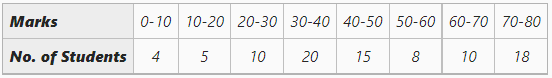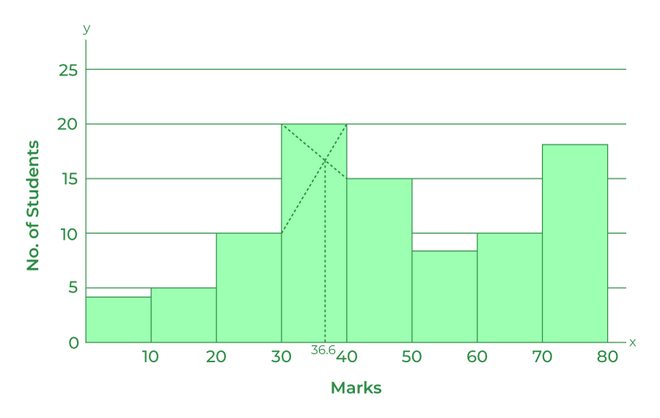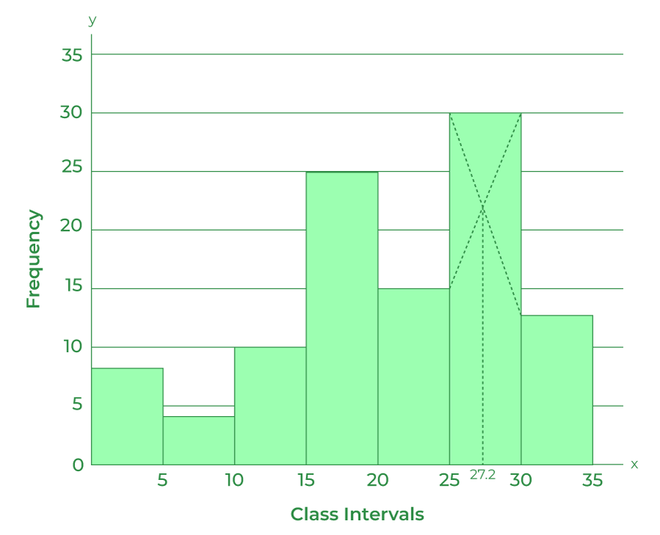GFG App
Open AppBrowser
Continue

# Calculation of Mode by Graphical Method

The word mode is derived from the French word ‘La Mode’, meaning anything that is in fashion or vogue. A measure of central tendency in statistical series that determines the value occurring most frequently in the given series is known as mode. In other words, the modal value of the series has the highest frequency in the given series. For example, if in a class of 100 students, 20 students have opted for Mathematics, 50 students have opted for IP, and the rest 30 have opted for Physical Education, then the modal opted subject of the class would be IP.

Mode is represented by the letter Z and can be calculated in three different series; viz., individual series, discrete series, and continuous series.

According to Kenny, “The value of the variable which occurs most frequently in a distribution is called the mode.”

According to Croxton and Cowden, “The mode may be regarded as the most typical of a series of values.”

#### Mode by Graphical Method

The mode for a given set of series can be located through a histogram. The steps to locate mode through Graph are as follows:

#### Step 1

Draw a histogram of the given set of series.

#### Step 2

The rectangle of the histogram with the greatest height will be the modal class of the series.

#### Step 3

Draw a line joining the top left corner/point of the rectangle of the modal class to the top left corner/point of the rectangle of the succeeding class.

#### Step 4

Similarly, draw a line joining the top right corner/point of the rectangle of the modal class to the top right corner/point of the rectangle of the preceding class.

#### Step 5

Draw a line perpendicular to the X-axis from the intersection point of the two lines drawn in the previous two steps.

#### Step 6

The point at which the perpendicular line cuts the X-axis is the modal value of the given series.

Example 1:

Determine mode of the following series, using the Graphic Method.#### Solution:Verification: The modal value can be verified with Inspection Method.

The formula for calculating Mode through Inspection Method is,= 30 + 6.67

= 36.67

#### Example 2:

Determine mode of the following series, using the Graphic Method.

Solution:Verification: The modal value can be verified with Inspection Method.

The formula for calculating Mode through Inspection Method is,= 25 + 2.27

= 27.27

My Personal Notes arrow_drop_up